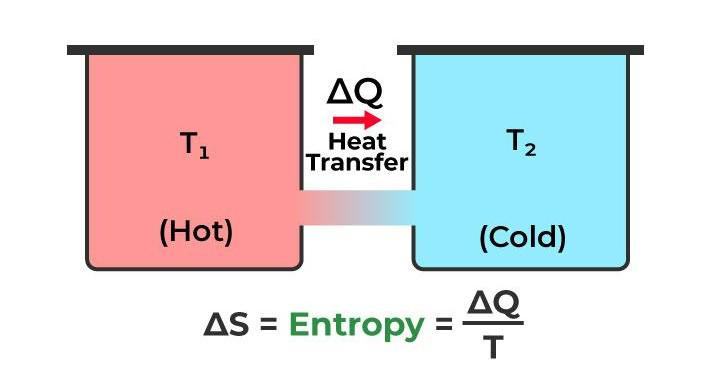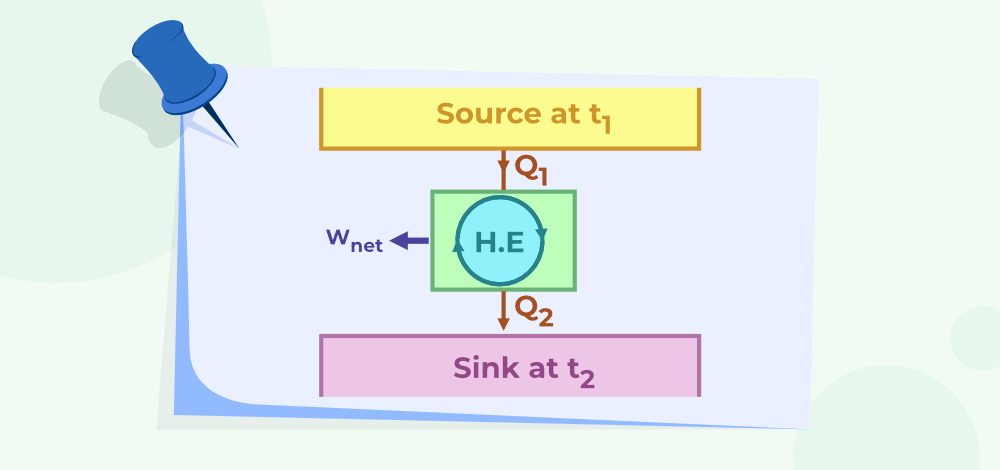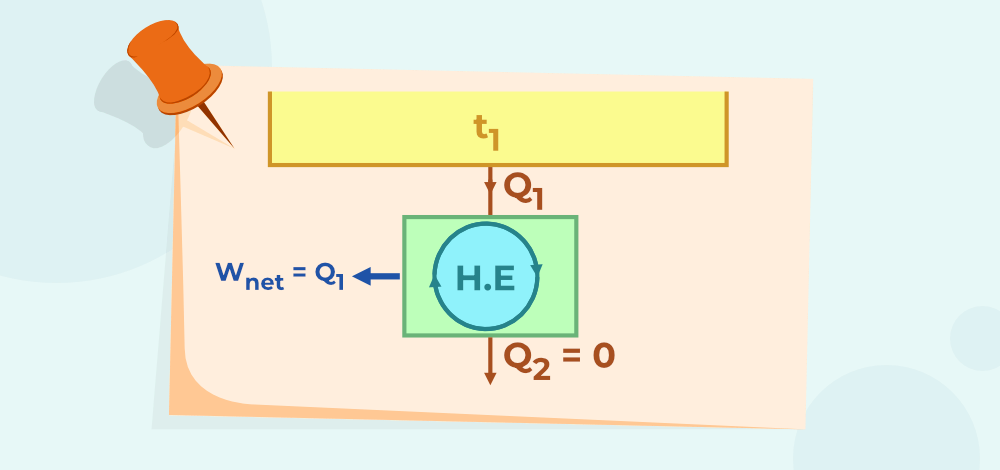GFG App
Open AppBrowser
Continue

# Second Law of Thermodynamics

Second law of thermodynamics defines that heat cannot move from a reservoir of lower temperature to a reservoir of higher temperature in a cyclic process. The second law of thermodynamics deals with the transfer of heat naturally from a hotter body to a colder body. Second Law of Thermodynamics is one of three Laws of Thermodynamics. The word “thermodynamics” comes from two root words: “thermo,” meaning heat, and “dynamic,” meaning power. Thus, the Laws of Thermodynamics are the Laws of “Heat Power. The First Law of Thermodynamics is commonly known as the Law of Conservation of Matter. While the Second Law of Thermodynamics is commonly known as the Law of Increased Entropy.

## What is the Second Law of Thermodynamics?

According to the second law of thermodynamics, any naturally occurring process will always cause the universe’s entropy (S) to increase. The law simply states that the entropy of an isolated system will never diminish over time.

The second law of thermodynamics states that the heat energy cannot transfer from a body at a lower temperature to a body at a higher temperature without the addition of energy.The overall entropy of a system and its surroundings remains constant in some instances where the system is in thermodynamic equilibrium or going through a reversible process. The Law of Increased Entropy is another name for the second law.

## Different Statements of Second Law of Thermodynamics

The second law of thermodynamics may be expressed in many specific ways, by different scientists at different times. Here are the Second Law of Thermodynamics statements:

### Kelvin-Planck Statement of Second Law of Thermodynamics

It’s difficult to turn all of the heat emitted by a heated body into work. The working material of a heat engine absorbs heat from a hot body, transforms a portion of it into work, and returns the remainder to the cold body. No engine can transform all of the heat from the source into work without wasting any heat. This indicates that a sink is required to get continuous work.

The following image shows the working of the heat engine.### Clausius Statement of Second Law of Thermodynamics

It is impossible to build a technology that can transmit heat from a colder body to a warmer body without wasting any energy. In other words, the refrigerator will not work unless the compressor is powered by an external source. Clausius’s assertion is used by heat pumps and refrigerators.

### Equivalence of Two Statements

A refrigerator, for example, can transfer a certain amount of heat from a cold body to a hot one without requiring any external energy. As a result, Clausius’ assertion is violated. Assume that an engine operating between the same hot and cold bodies absorbs heat from the hot body, transforms a portion W into work, and then transfers the remaining heat to the cold body.

The engine does not break the second law of thermodynamics on its own. When the engine and the refrigerator operate together, they create a mechanism that absorbs all of the heat from the hot body and transforms it into work without sacrificing any of the cold body’s heat. The Kelvin-Planck assertion is violated. As a result, we may conclude that the two versions of the second law of thermodynamics are equivalent in every way.

## Second Law of Thermodynamics Equation

The second law of thermodynamics is expressed mathematically as;

ΔSuniv > 0

Here,
ΔSuniv is a change in the universe’s entropy.

Let’s look at the definition of entropy and how it relates to the second rule of thermodynamics. The entropy of a system is defined as the number of changes it has undergone from its prior condition to its current state. As a result, entropy is usually expressed as a change in the entropy of the system indicated by ∆S. If the value of entropy at a given state of the system must be measured, then the previously chosen state of the system is assigned a value of zero entropy.

An isentropic process is one in which the system’s entropy remains constant throughout time. The entropy of the system in both the start and end states remains constant during the isentropic process. As a result, the value of S=0 during the isentropic process. It is only ideal process that the reversible isentropic process happens. In practice, anytime a change in the state of the system occurs, the entropy of the system rises.

## Perpetual Motion Machine of Second Kind

Perpetual motion machines are defined as devices that work while connecting with only one heat reservoir. These devices are considered to violate the second law of thermodynamics. These machines do not exist in the real life and only exist theoretically.

We know that a heat engine interacts with two heat reservoirs at different temperatures to produce work in a cycle. The differences in the temperature of the two reservoirs result in the work being produced until the temperature in both reservoirs is equalized.

The following image shows a perpetual machine.## Application of the Second Law of Thermodynamics

Here are the Applications and Uses of thermodynamic’s Second Law:

• The law states that heat always moves from a body that is warmer to a body that is colder. All heat engine cycles, including Otto, Diesel, etc., as well as all working fluids employed in the engines, are covered by this rule. Modern automobiles have advanced as a result of this law.
• Refrigerators and heat pumps that use the Reversed Carnot Cycle are other examples of how this concept is applied. You must provide external work if you want to transfer heat from a body with a lower temperature to a body with a greater temperature. Unlike the Reversed Carnot Cycle, which uses work to move heat from a lower-temperature reservoir to a higher-temperature reservoir, the original Carnot Cycle uses heat to produce work.

## Limitations of Second Law of Thermodynamics

Lets now read out the shortcomings or drawbacks of the Second Law of Thermodynamics:

• The second law of thermodynamics is a concept that limits the occurrence of many processes that, despite being permitted by other physical laws, we know from experience does not occur. For instance, water in a glass that is at ambient temperature never spontaneously cools to create ice cubes, which would release energy into the environment.
• Suggesting that the universe will end in a state of “heat death,” in which everything is the same temperature, the second rule also predicts the end of the universe. When everything is at the same temperature, there can be no work done and all of the energy is squandered as random atom and molecule motion, which is the most extreme level of disorder.

## Solved Examples on the Second Law Of Thermodynamics

Example 1: If a heat pump works for 600 J and removes 800 J of heat from the low-temperature reservoir. What is the amount of heat delivered to a higher-temperature reservoir?

Solution:

Given,
W = 600 J
QC = 800 J
QH  =?

Now, according to the laws of thermodynamics.

QH = W + QC

QH = 600 J + 800 J

QH = 1400 J

Thus, the heat delivered to the higher temperature reservoir is 1400 J.

Example 2: Find the work done by the heat pump if the heat pump removes 1000 J of heat from high-temperature and delivers 400 J to the low-temperature reservoir.

Solution:

Given,
W =?
QC = 400 J
QH  = 1000 J

Now, according to the laws of thermodynamics.

QH = W + QC

1000 J = W + 400 J

W = 1000 – 400

W = 600 J

Thus, the work done by the heat pump is 600 J

Example 3: For a reversible heat engine the heat received is 1400 kJ at a temperature of 500K. If the surrounding temperature is 200K then the available amount of heat energy for doing work is?

Solution:

Q1 = 1400 KJ

T1 = 500K

T2 = 200 K

ΔS = Q1 / (T1 + T2)

ΔS = 1400 / (500 + 200)

ΔS = 2 KJ/K

The availability of heat energy for doing work is,

H = Q1 – T2(ΔS)

H = 1400 – 200(2)

H = 1000 KJ

Example 4: For a reversible heat engine the heat received is 1200 KJ at a temperature of 400K and ΔS = 2 KJ/K, then find the temperature of the surrounding.

Solution:

Q1 = 1200 KJ

T1 = 400K

T2 =?

ΔS = 2 KJ/K

we know that,

ΔS = Q1 / (T1 + T2)

2 = 1200 / (400 + T2)

400 + T2 = 600

T2 = 600 – 400 = 200 K

Thus, the temperature of the surrounding is 200 K.

## FAQs on the Second Law of Thermodynamics

### Question 1: State Second Law of Thermodynamics.

The second law of thermodynamics states that the heat energy cannot transfer from a body at a lower temperature to a body at a higher temperature without the addition of energy.

### Question 2: State the second law of thermodynamics Clausius statement.

According to Clausius’s statement, “It is impossible to construct a device that operates in a cycle and produces no effect other than the transfer of heat from a cooler body to a hotter body.”

### Question 3: State the second law of thermodynamics Planks statement.

According to Kelvin-Planck’s statement, ” It is impossible to build a heat engine that takes heat from the hot reservoir (QH) and converts all the energy into useful external work without losing heat to the cold reservoir (QC).”

### Question 4: What is the difference between the First and Second Law of Thermodynamics?

First Law of Thermodynamics states that Energy cannot be created or destroyed. However, the Second Law of Thermodynamics states that for an spontaneous process the antropy of the system always increases.

### Question 5: What are applications of the Second Law of Thermodynamics?

Here are the Applications and Uses of thermodynamic’s Second Law:

• The law states that heat always moves from a body that is warmer to a body that is colder. All heat engine cycles, including Otto, Diesel, etc., works on this law.
• Refrigerators and heat pumps that use the Reversed Carnot Cycle are another example of working of Second Law of Thermodynamics.

### Question 6: What is Entropy?

Entropy is the measure of thermal energy of the system per unit temperature which is not required for doing useful work.